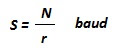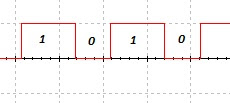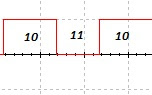## Audio

[Audio][slideshow]

The two most common/confused words in digital communication – Bit rate and Baud rate. Generally, communication is concerned with transmission of data. In digital communication, there are two entities that are needed to carry out communication – the data to be transmitted and the signal over which the data is transmitted. Now, we have two entities to be worried about – the data and the signal. The most common misconception is that most people think both travel at the same speed! – NO!

### The difference:

Digital data is very different from digital signal. The process of converting digital data to digital signal is called as line coding.Now, to discriminate between data and signal, data is what we need to send. But signal is what we can send. So, signal is the carrier which carries data.Also, keep in mind that the smallest entity of the data, that can represent a piece of information is called data element and shortest meaningful unit of a signal is called signal element. Consider this as in the following scenario – Consider a train. Each carriage is a signal element. Each passenger inside the train is a data element. The train as a whole is a signal and all passengers together represent a data.

### Data rate and Signal rate:

• Data rate – Number of data elements transmitted per second.
• Signal rate – Number of signal elements transmitted per second.

Now, the unit of data rate is bit rate. And the unit of signal rate is pulse rate/ modulation rate/ baud rate or simply baud. From the previous example, we can see that, a carriage in a train can carry more than one person. So, if you consider the number of person is more than one per carriage, you can say that bit rate is greater than baud rate for the signal.

### Calculating the baud rate:

Baud rate is calculated using the below formula.here, N is the bit rate and r is the number of data elements carried by each signal element. Here r must be as great as possible for better efficiency. (Stuff more people in a carriage :P)

From the above text, it is clearly inferred that the bit rate must be greater than the baud rate for higher efficiency. The aim is to transmit as many data element as possible in a signal element. There are different methods to do this which are collectively called as line coding schemes.Some of the popular line coding schemes are: Non-return to zero (NRZ), Manchester, Alternate mark inversion (AMI) and also multi level schemes are available.

### Few Examples:

Consider the below figures.Here, the data rate(bitrate) and signal rate(baud) are same and r is one. One data element rides on one signal element (analogous to one person per carriage in a train).And in this image, you can see that there are two data elements transmitter per signal element. In other words, the bit rate is higher than the baud rate. (Analogous to two passengers per carriage in a train) and here r is two. Hence baud rate is one half of bit rate.
And the next time, when you define the baud rate – its number of signal elements per second and not number of bits per second!

[Lights][grids]